### 2021 travel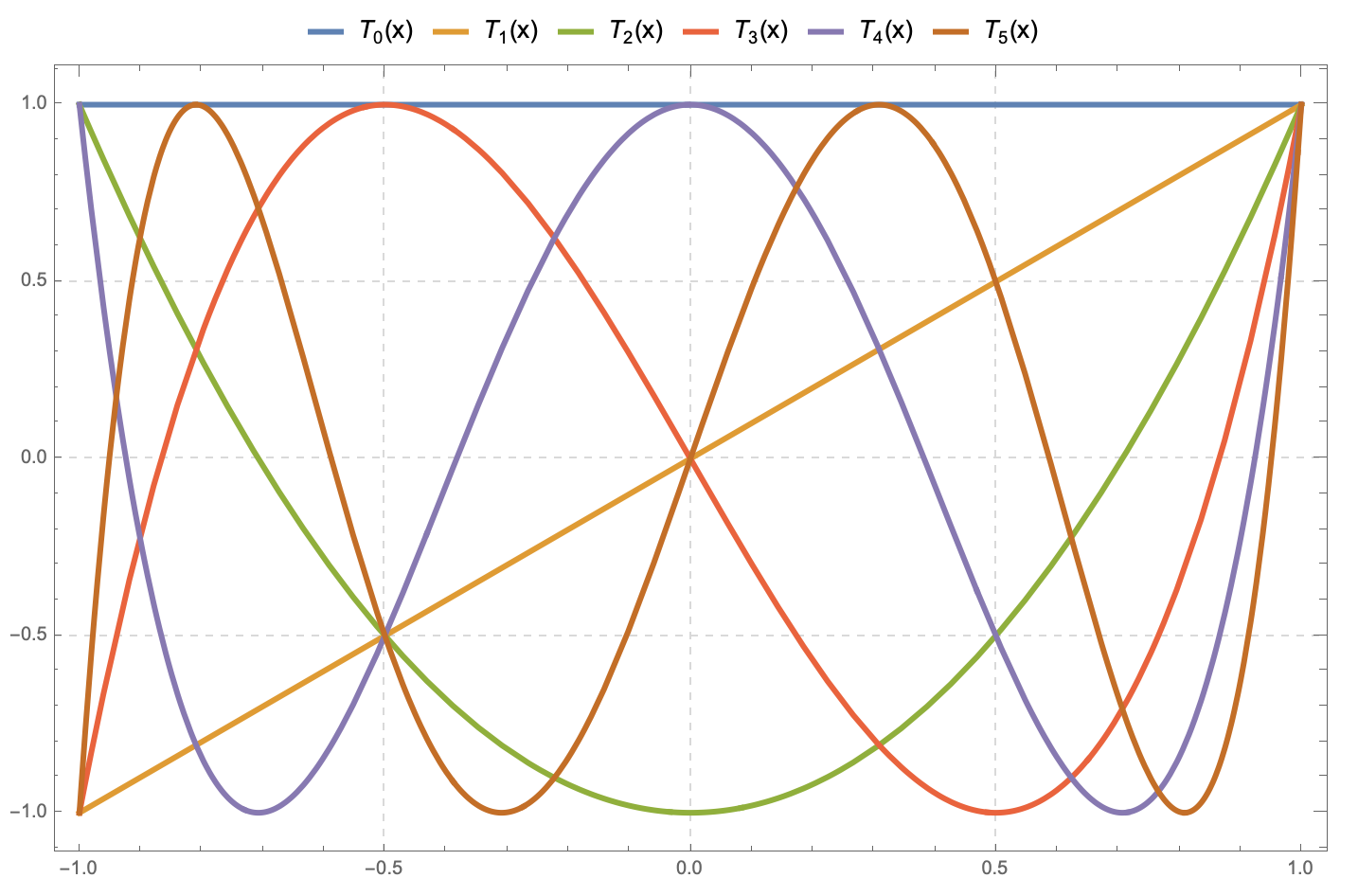### MATH 7310 • Real Analysis and Linear Spaces I

[Spring 2020 semester]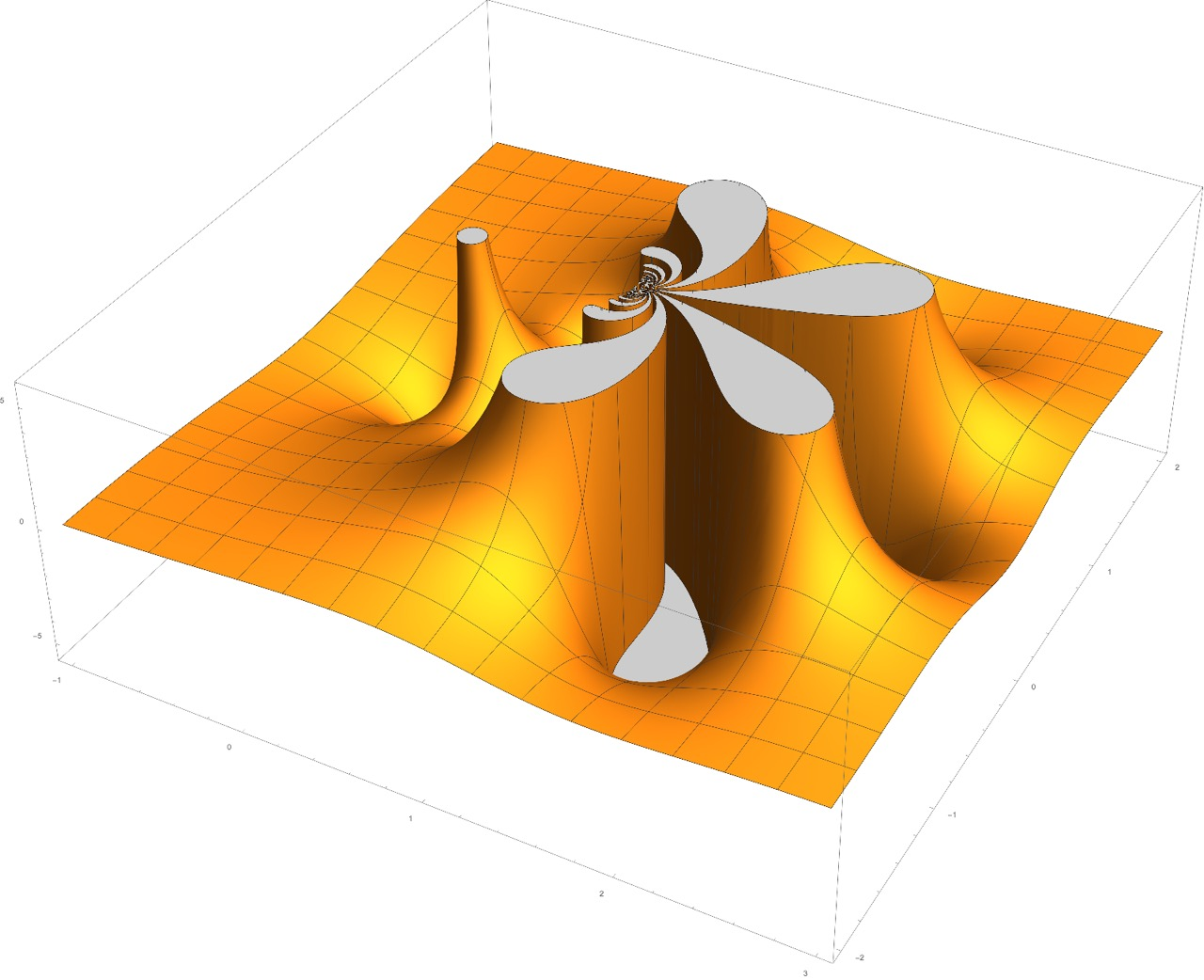### MATH 3340 • Complex Variables with Applications

[Spring 2020 semester]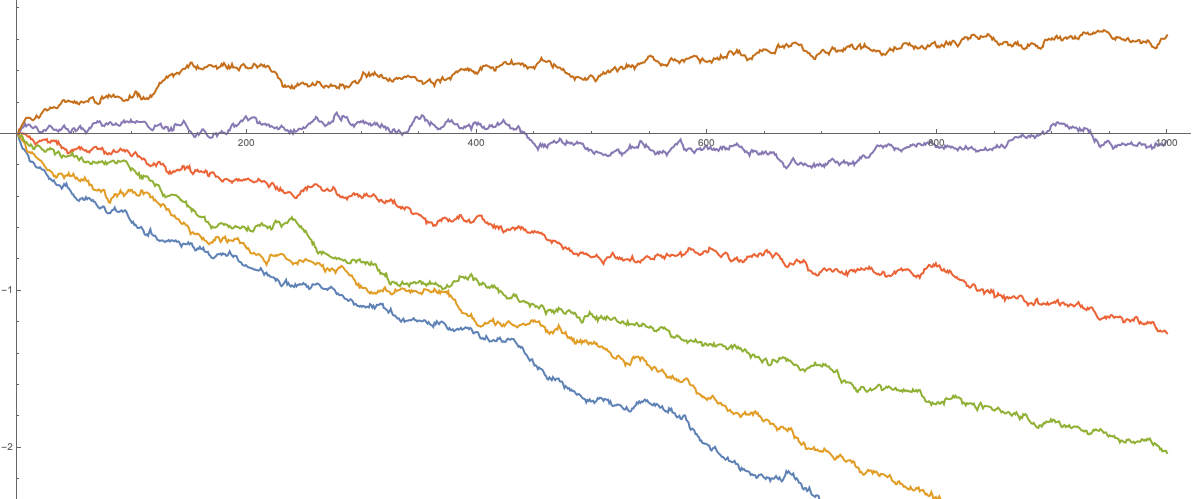### Parameter symmetry in perturbed GUE corners process and reflected drifted Brownian motions

[2019/12/17]

The perturbed GUE corners ensemble is the joint distribution of eigenvalues of all principal submatrices of a matrix $G+\mathrm{diag}(\mathbf{a})$, where $G$ is the random matrix from the Gaussian Unitary Ensemble (GUE), and $\mathrm{diag}(\mathbf{a})$ is a fixed diagonal matrix. We introduce Markov transitions based on exponential jumps of eigenvalues, and show that their successive application is equivalent in distribution to a deterministic shift of the matrix. This result also leads to a new distributional symmetry for a family of reflected Brownian motions with drifts coming from an arithmetic progression.

The construction we present may be viewed as a random matrix analogue of the recent results of the first author and Axel Saenz.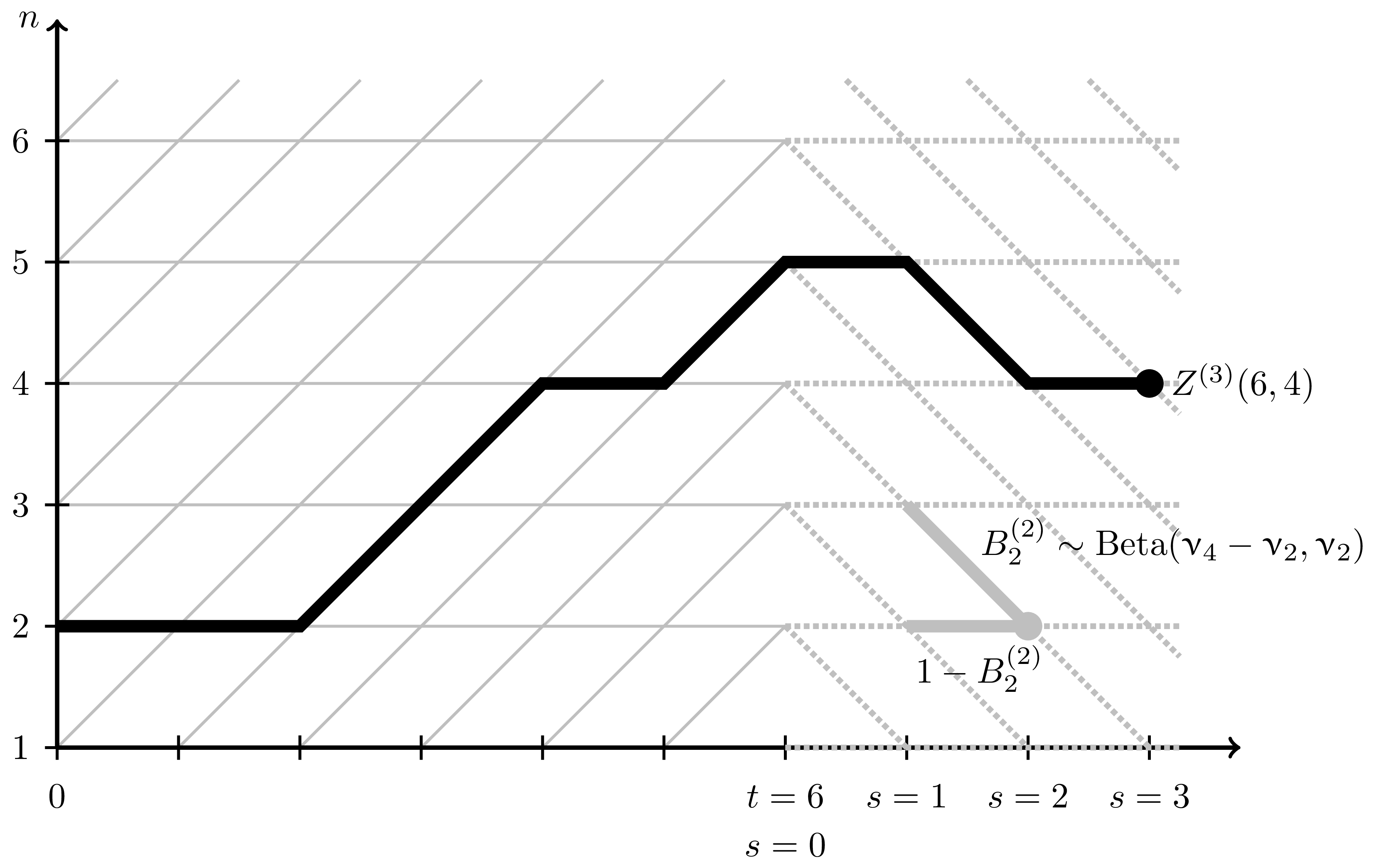### Parameter permutation symmetry in particle systems and random polymers

[2019/12/12]

Many integrable stochastic particle systems in one space dimension (such as TASEP - Totally Asymmetric Simple Exclusion Process - and its various deformations, with a notable exception of ASEP) remain integrable when we equip each particle $x_i$ with its own jump rate parameter $\nu_i$. It is a consequence of integrability that the distribution of each particle $x_n(t)$ in a system started from the step initial configuration depends on the parameters $\nu_j$, $j\le n$, in a symmetric way. A transposition $\nu_n \leftrightarrow \nu_{n+1}$ of the parameters thus affects only the distribution of $x_n(t)$. For $q$-Hahn TASEP and its degenerations (namely, $q$-TASEP and beta polymer) we realize the transposition $\nu_n \leftrightarrow \nu_{n+1}$ as an explicit Markov swap operator acting on the single particle $x_n(t)$. For beta polymer, the swap operator can be interpreted as a simple modification of the lattice on which the polymer is considered. Our main tools are Markov duality and contour integral formulas for joint moments.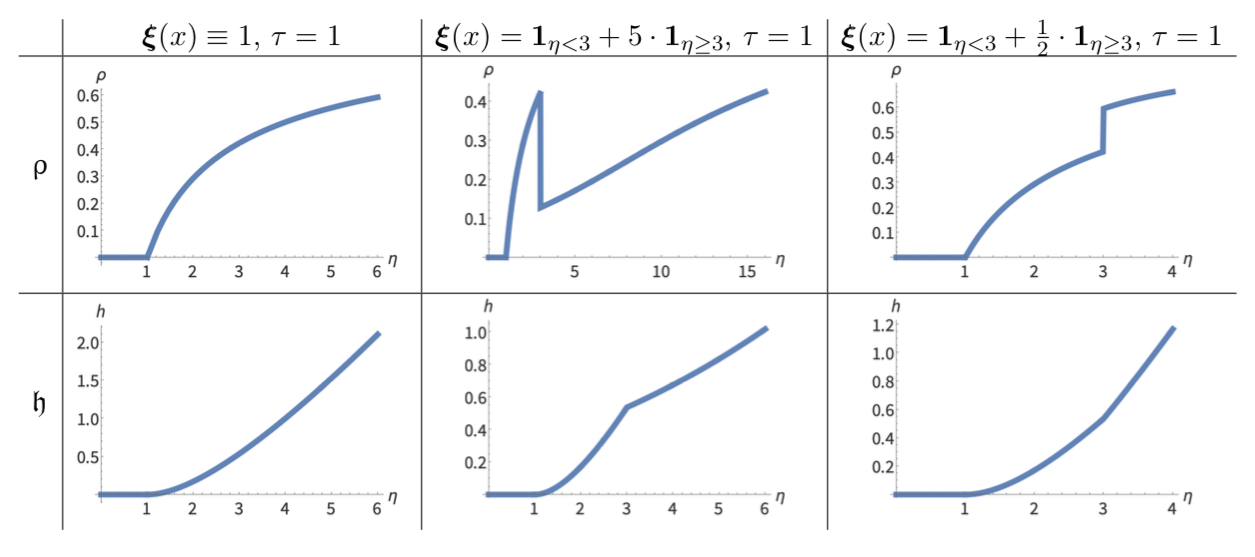### PushTASEP in inhomogeneous space

[2019/10/20]

We consider the PushTASEP (pushing totally asymmetric simple exclusion process, also sometimes called long-range TASEP) with the step initial configuration evolving in an inhomogeneous space. That is, the rate of each particle’s jump depends on the location of this particle. We match the distribution of the height function of this PushTASEP with Schur processes. Using this matching and determinantal structure of Schur processes, we obtain limit shape and fluctuation results which are typical for stochastic particle systems in the Kardar-Parisi-Zhang universality class. PushTASEP is a close relative of the usual TASEP. In inhomogeneous space the former is integrable, while the integrability of the latter is not known.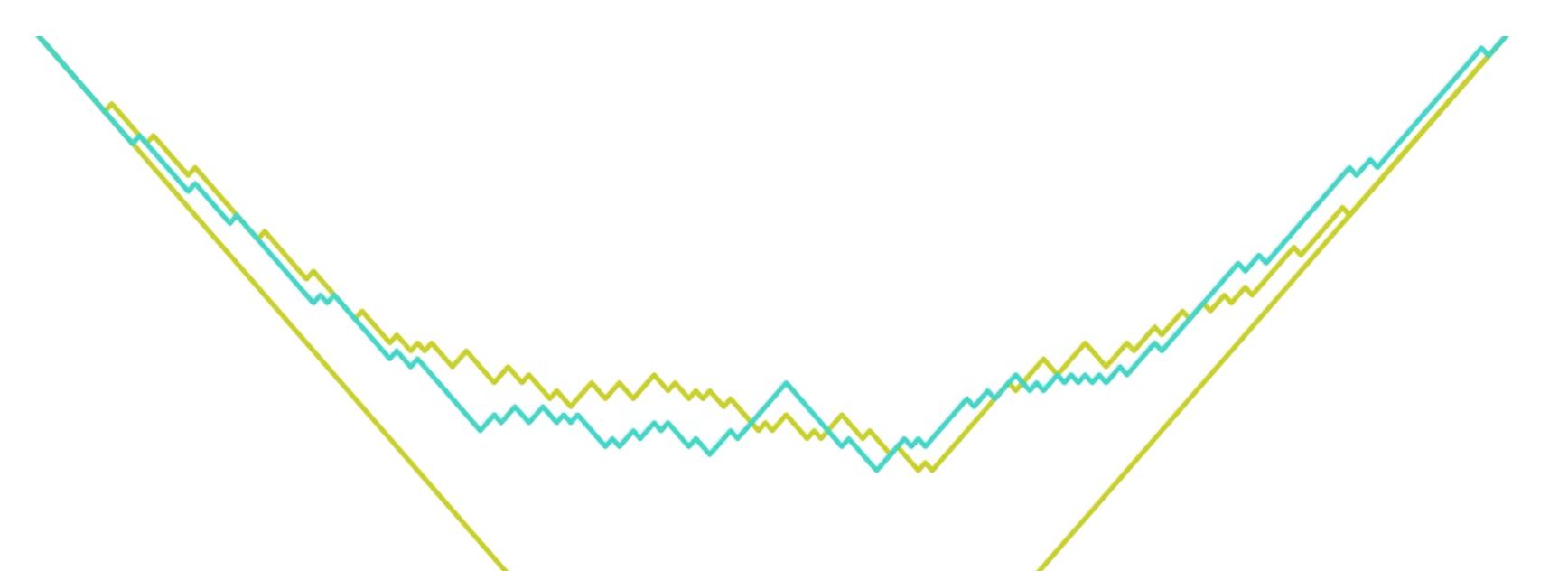### Mapping TASEP back in time

We obtain a new relation between the distributions $\mu_t$ at different times $t ≥ 0$ of the continuous-time TASEP (Totally Asymmetric Simple Exclusion Process) started from the step initial configuration. Namely, we present a continuous-time Markov process with local interactions and particle-dependent rates which maps the TASEP distributions μt backwards in time. Under the backwards process, particles jump to the left, and the dynamics can be viewed as a version of the discrete-space Hammersley process. Combined with the forward TASEP evolution, this leads to a stationary Markov dynamics preserving $\mu_t$ which in turn brings new identities for expectations with respect to $\mu_t$. Based on a joint work with Axel Saenz.

Note that the original keynote presentation contained videos which are not included in the PDF download.